##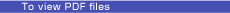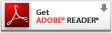Special Feature: Silicon Photonic Technologies Leading the Way to a New Generation of Telecommunications

# Entanglement Generation Using Silicon Wire Waveguide

## Abstract

Generating high-purity entangled photon pairs is an important step toward quantum information processing using photons. In this article, we describe the world’s first ever experiment on generating entanglement using a spontaneous parametric process in a silicon wire waveguide.

† NTT Basic Research Laboratories Atsugi-shi, 243-0198 Japan

## 1. Introduction

In recent years, information processing using the principles of quantum mechanics, including quantum cryptography and quantum computation, has been studied intensively. Quantum entanglement is an essential resource for quantum information processing. In particular, an entangled photon pair , which is an entangled state of photons, has been investigated as a way of distributing entanglement over long distances. In this article, we describe the world’s first ever experiment on generating entangled photon pairs using a silicon wire waveguide , .

## 2. Time-bin-entangled photon pair

An entangled photon pair is a state where two photons show a correlation that cannot be explained by the classical theory. For example, a polarization-entangled photon pair is a state in which the polarization state of each photon is not fixed, but the relationship between the polarization states of the two photons is predetermined . On the other hand, a time-bin-entangled state is based on a time-bin qubit, which is a superposition of two quantum states of photons in two distinguishable temporal positions. In a similar manner to that for polarization-entangled photons, we can describe a time-bin-entangled photon pair as being a state in which the temporal position of each photon is not fixed, but the two photons are always found in the same time slot. Here, we call the photons of an entangled pair the signal photon and the idler photon. The quantum state of a time-bin-entangled photon pair is given by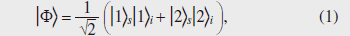wherexy denotes the quantum state where there is a photon in the xth time slot and in mode y (=s: signal, i: idler).

In fact, we can get the situation in which the temporal position of each photon is not fixed, but the two photons are always found in the same time slot using two classically correlated photons. However, with classically correlated photons, the correlation between the measurement outcomes vanishes if we measure the photons using a basis that is not orthogonal to the original basis (for example, a polarization measurement that discriminates ±45° linear polarization is non-orthogonal to a horizontal/vertical polarization measurement). An example of a measurement that is non-orthogonal to the measurement that discriminates the states1y and2y is one that discriminates whether the photon is in a superposition of1y and2y with a relative phase of 0 or π. This corresponds to a measurement that determines whether the state is one of the states+y ory defined below.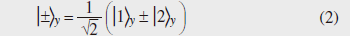Using the states defined in Eq. (2), we can rewrite Eq. (1) as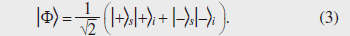This equation clearly shows that the correlation of the measurement outcomes for two photons is still preserved even when we use a non-orthogonal measurement basis. This is a very important characteristic of an entangled photon pair, which cannot be achieved using two classically correlated photons.

## 3. Quantum key distribution using entangled photon pairs

We can implement various quantum information tasks using the peculiar characteristics of entangled photons explained in the previous section. Here, as an example of such a quantum information task, we describe quantum key distribution (QKD), where a secret key for cryptography is distributed between two distant parties. The configuration, in which a key is established between Alice and Bob, is shown in Fig. 1. We prepare many entangled pairs and send the signal and idler photons to Alice and Bob, respectively, over conventional optical transmission lines such as optical fibers. Both Alice and Bob are equipped with two measurement setups that correspond to two non-orthogonal measurements. Here, we call the measurement that discriminate states1y and2y the “X basis” and that for+y ory the “Y basis”. Alice and Bob measure each incoming photon using a basis randomly chosen from X and Y. They record the measurement outcome and the basis used for each measurement. After measuring all the photons, they disclose which basis they used for each measurement through a conventional communication line. Note that they do not disclose the measurement outcomes. Then, by extracting the events where their measurement bases (accidentally) coincided, they can share correlated measurement outcomes without disclosing them. As a result, Alice and Bob can share a random bit string by assigning, for example, the outcomes (1y,+y ) as bit 0 and (2y ,y ) as bit 1. This bit string can be used as a key for one-time-pad cryptography.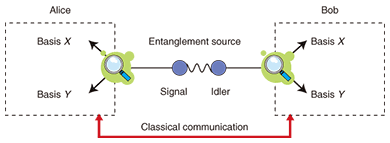Fig. 1. QKD using entangled photon pairs.

It is fundamentally impossible to eavesdrop on the key created by QKD, so we can achieve unconditionally secure communication by using QKD. Intuitively, the security originates from the fact that any two classically correlated photons cannot fully mimic the characteristics of entangled photon pairs (of course, very careful treatment is required to prove the unconditional security of any QKD protocol). Currently, secret communication based on QKD is expected to provide ultimate network security for future communication networks.

## 4. Entanglement generation using silicon wire waveguide

We generated 1.5-μm-band entangled photon pairs using a silicon wire waveguide . A silicon wire waveguide is a single-mode, single-crystal silicon waveguide whose core diameter is typically 400 nm wide and 200 nm thick. Thanks to this extremely small core, the optical power density in the waveguide is very high, and as a result, we can observe a huge nonlinear effect. The nonlinear coefficient of the waveguide used in our experiment was five orders of magnitude higher than that of a conventional silica fiber. Since silicon is an inversion symmetric material, we can use it for a third-order nonlinearity experiment. In the present experiment, we generated time-bin-entangled photon pairs by spontaneous four-wave mixing (SFWM), which is one of the third-order nonlinear effects.

When we input a 1.5-μm pump light whose angular frequency is given by ωp into a silicon wire waveguide, we can generate a photon pair with angular frequencies of ωs and ωi through SFWM. The following relationship exists between ωp, ωs, and ωi.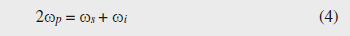This equation implies that two pump photons are annihilated and a signal-idler photon pair is created. Since the two photons are created simultaneously, we can generate a temporally correlated photon pair state, in which two photons are always observed in the same time slot (for example,1s1i ).

Thus, we can obtain a temporally correlated state, but it is still not entangled, as shown by Eq. (1). To generate an entangled state, we use a double pulse as a pump. Here, the phase difference between two pump pulses is fixed, so the first and second pulses probabilistically create pair states1s1i and2s2i, respectively. By using a relatively low pump power, we can make the probability of simultaneous generation of both pair states very low. As a result, we can obtain a coherent superposition of the1s1i and2s2i states, which is a time-bin-entangled state given by Eq. (1).

This technique enables us to make a simple, stable entanglement source in the 1.5-μm band. In addition, the use of a silicon waveguide enables us to avoid noise photons caused by spontaneous Raman scattering, which has been a serious problem for entanglement sources based on SFWM in an optical fiber .

## 5. Experiment

We generated time-bin-entangled photon pairs using the setup shown in Fig. 2. We injected 1551.1-nm double pulses into a silicon wire waveguide. The waveguide used in the experiment was 460 nm wide, 220 nm thick, and 1 cm long, and the waveguide loss was approximately 1 dB. The SFWM process inside the waveguide generated the quantum state shown by Eq. (1). The photons generated from the waveguide were first passed through a fiber Bragg grating to suppress the pump photons and input into an arrayed waveguide grating to separate the signal and idler photons. The wavelengths of the signal and idler photons were 1547.9 and 1554.3 nm, respectively. Each photon was passed through an optical bandpass filter to further suppress the residual pump photons, and then launched into a 1-bit delayed interferometer, which is an unbalanced Mach-Zehnder interferometer whose path difference corresponds to the time interval between the two pump pulses. The photons output from the interferometers were detected by single-photon detectors.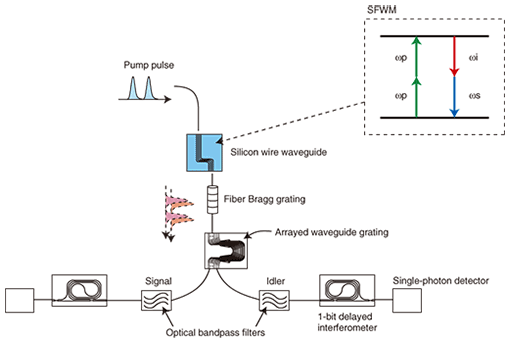Fig. 2. Setup for generating time-bin-entangled photon pairs.

When a photon whose state spans two time slots is launched into the 1-bit delayed interferometer, the single-photon detector connected to the interferometer output could detect the photon in any one of three time slots. Photon detection in the second time slot means that the input state is projected to a state given by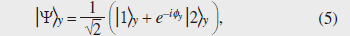where φy is the phase difference induced in the interferometer. Thus, we can implement measurement bases that correspond to+y andy described above, by setting φy to 0 and –π, respectively.

To confirm the generation of time-bin entanglement, we set the phase difference of the signal interferometer to 0 (φs = 0) and observed the coincidence counts of two photons as a function of idler interferometer phase difference φi. The results are shown by squares in Fig. 3. We observed a clear sinusoidal modulation of the coincidence counts, which indicates that the measurement results for the signal and idler photons were correlated. The waveform had peak values at φi = 2 n π (n: integer). This means that the coincidence rate was maximum when the signal and idler photons were both projected to+y, reflecting the characteristics suggested by Eq. (3).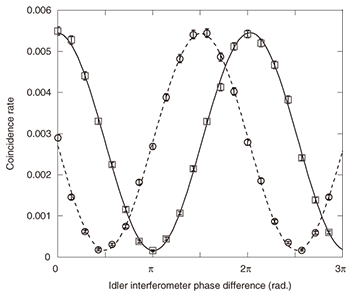Fig. 3. Results of coincidence measurements between the signal and idler photons. Squares and circles are the coincidence fringes obtained for a signal interferometer phase differences of 0 and –π/2, respectively.

We then set φs = –π/2, which let us implement a non-orthogonal basis for measuring signal photons, and measured the coincidence fringe. The results (circles in Fig. 3) clearly show that the measurement results for two photons were again correlated even with the non-orthogonal basis. Thus, we successfully confirmed the generation of an entangled state. The visibilities of the coincidence fringes were 96.3% (φs = 0) and 95.2% (φs = –π/2), implying that we obtained high-purity entanglement in the 1.5-μm band.

## 6. Conclusion

NTT has performed the world’s first ever entanglement generation experiment using a silicon wire waveguide. This technique is expected to provide a practical and high-purity entanglement source in the 1.5-μm telecommunication band, which will enable us to achieve quantum communication over optical fibers in the future.

## References

 H. Takesue, “Generation of polarization-entangled photon pairs in 1.5-μm telecommunication band,” NTT Technical Review Vol. 3, No. 12, pp. 52–60, 2005. https://www.ntt-review.jp/archive/ntttechnical.php?contents=ntr200512052.pdf H. Takesue, Y. Tokura, H. Fukuda, T. Tsuchizawa, T. Watanabe, K. Yamada, and S. Itabashi, “Entanglement generation using silicon wire waveguide,” Appl. Phys. Lett., Vol. 91, No. 20, 201108, 2007. K. Harada, H. Takesue, H. Fukuda, T. Tsuchizawa, T. Watanabe, K. Yamada, Y. Tokura, and S. Itabashi, “Generation of high-purity entangled photon pairs using silicon wire waveguide,” Opt. Express, Vol. 16, No. 25, pp. 20368–20373, 2008. T. Tsuchizawa, K. Yamada, H. Fukuda, T. Watanabe, J. Takahashi, M. Takahashi, T. Shoji, E. Tamechika, S. Itabashi, and H. Morita, “Microphotonics Devices Based on Silicon Microfabrication Technology,” IEEE J. of Select. Topics Quant. Electron., Vol. 11, No. 1, pp. 232–240, 2005. H. Takesue and K. Inoue, “1.5-μm band quantum-correlated photon pair generation in dispersion-shifted fiber: suppression of noise photons by cooling fiber,” Opt. Express, Vol. 13, No. 20, pp. 7832–7839, 2005.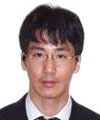Hiroki Takesue Senior Research Scientist, Quantum Optical State Control Research Group, NTT Basic Research Laboratories. He received the B.E., M.E., and Ph.D. degrees in engineering science from Osaka University in 1994, 1996, and 2002, respectively. He joined NTT Laboratories in 1996, where he engaged in research on lightwave frequency synthesis, optical access networks using wavelength division multiplexing, and quantum communication. From 2004 to 2005, he was a Visiting Scholar at Stanford University, California, USA. He is a member of IEEE and the Japan Society of Applied Physics (JSAP). Ken-ichi Harada Research Associate, Quantum Optical State Control Research Group, NTT Basic Research Laboratories. He received the B.S., M.S., and Ph.D. degrees in physics from Kumamoto University in 2001, 2003, and 2006, respectively. He is currently engaged in experimental studies on quantum communication systems using silicon photonic waveguides. He is a member of the Physical Society of Japan (JPS) and JSAP. Yasuhiro Tokura Executive Manager, Optical Science Laboratory, NTT Basic Research Laboratories. He received the B.S., M.S., and Ph.D. degrees in arts and science from the University of Tokyo in 1983, 1985, and 1998, respectively. Since 1985, he has been with NTT Laboratories, where he has engaged in the research on condensed-matter physics and transport theory in low-dimensional systems. He is a member of JPS and JSAP. Hiroshi Fukuda Senior Research Engineer, Nano Silicon Technology Research Group, NTT Microsystem Integration Laboratories. He received the B.E. and M.E. degrees in nuclear engineering from Tohoku University, Miyagi, in 1993 and 1995, respectively. He joined NTT Laboratories in 1995 and has since been engaged in research on microphotonic devices. He is a member of the Institute of Electronics, Information and Communication Engineers (IEICE) of Japan. Tai Tsuchizawa Senior Research Engineer, Nano Silicon Technology Research Group, NTT Microsystem Integration Laboratories. He received the Ph.D. degree from the Department of Pure and Applied Sciences, the University of Tokyo, in 1990. He joined NTT in 1990 and engaged in studies on ECR plasma and its application to etching processes for microfabrication. He is currently engaged in studies on fabrication technology for silicon-based optoelectronic devices. He is a member of JSAP. Toshifumi Watanabe Senior Research Engineer, Nano Silicon Technology Research Group, NTT Microsystem Integration Laboratories. He received the B.S. and M.S. degrees in applied physics from Tokyo Institute of Technology in 1982 and 1984, respectively. He is currently working in NTT Laboratories, where he is engaged in layout processing studies for electron-beam pattern writers and for computer-aided design data modulation for large-scale integration and photonic circuits. His current research interests include robust processing algorithms for very complex pattern layouts. Koji Yamada Senior Research Engineer, Distinguished Technical Member, Nano Silicon Technology Research Group, NTT Microsystem Integration Laboratories. He received the B.E., M.E., and Ph.D. degrees in nuclear engineering from Kyushu University, Fukuoka, in 1986, 1988, and 2003, respectively. He has been engaged in theoretical and experimental studies on beam dynamics in electron synchrotrons. Recently, he has been engaged in research on silicon-based nanophotonic structures. He is a member of JSAP, the Atomic Energy Society of Japan, the Particle Accelerator Society of Japan, IEICE, and IEEE. Sei-ichi Itabashi Senior Research Engineer, Supervisor, Nano Silicon Technology Research Group Leader, NTT Microsystem Integration Laboratories. He received the B.S. and M.S. degrees in physics from Tohoku University, Miyagi, in 1982 and 1984, respectively, and the Ph.D. degree from Hokkaido University in 1998. He joined Nippon Telegraph and Telephone Public Corporation (now NTT) in 1984. He has worked on the development of X-ray and extreme-ultraviolet sources for X-ray lithography in NTT Laboratories. He has also engaged in theoretical and experimental studies on highly reflective X-ray mirrors and multilayers. He is currently engaged in studies on silicon photonics technology. He is a member of JSAP.

↑ TOP